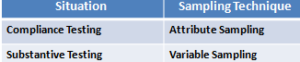# Sampling-Point to Remember

Point to remember for CISA exam-Sampling:

(1)In any given scenario, statistical sampling is to be used when the probability of error must be objectively quantified (i.e no subjectivity is involved).

(2)In any given scenario, following sampling is best suitable for compliance and substantive testing:(3)In any given scenario, best sampling technique where fraud is being suspected is Discovery Sampling.

(4)A higher confidence coefficient will result in the use of a larger sample size. In other way, higher sample size will give higher confidence coefficient.

(5)In any given scenario, when internal controls are strong, confidence coefficient /sample size may be lowered.

(6)In any given scenario, statistical sampling minimizes the detection risk.